# 1. 从感知机到神经网络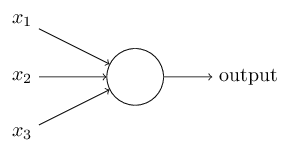$sign(z)= \begin{cases} -1& {z<0}\\ 1& {z\geq 0} \end{cases}$

1）加入了隐藏层，隐藏层可以有多层，增强模型的表达能力，如下图实例，当然增加了这么多隐藏层模型的复杂度也增加了好多。2）输出层的神经元也可以不止一个输出，可以有多个输出，这样模型可以灵活的应用于分类回归，以及其他的机器学习领域比如降维和聚类等。多个神经元输出的输出层对应的一个实例如下图，输出层现在有4个神经元了。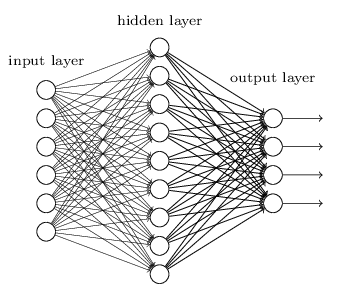3） 对激活函数做扩展，感知机的激活函数是sign(z),虽然简单但是处理能力有限，因此神经网络中一般使用的其他的激活函数，比如我们在逻辑回归里面使用过的Sigmoid函数，即：$f(z)=\frac{1}{1+e^{-z}}$

# 2. DNN的基本结构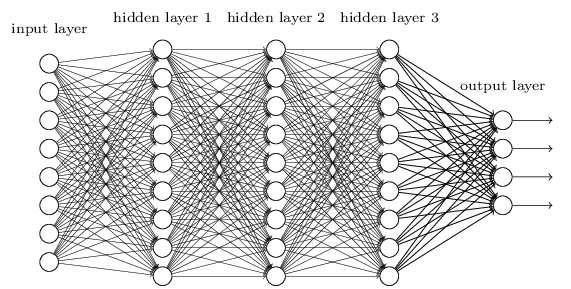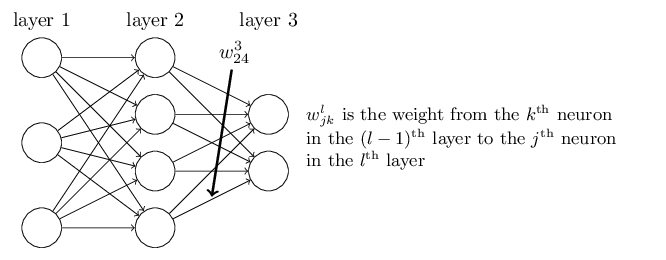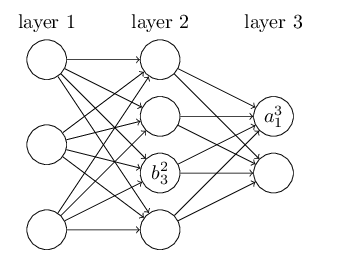# 3. DNN前向传播算法数学原理$a_1^2=\sigma(z_1^2) = \sigma(w_{11}^2x_1 + w_{12}^2x_2 + w_{13}^2x_3 + b_1^{2})$

$a_2^2=\sigma(z_2^2) = \sigma(w_{21}^2x_1 + w_{22}^2x_2 + w_{32}^2x_3 + b_2^{2})$

$a_3^2=\sigma(z_3^2) = \sigma(w_{31}^2x_1 + w_{32}^2x_2 + w_{33}^2x_3 + b_3^{2})$

# 4. DNN前向传播算法

1） 初始化$a^1 = x$

2) for l = 2 to L, 计算：$a^l = \sigma(z^l) = \sigma(W^la^{l-1} + b^l)$